# 2 Electromagnetic waves

## 2 Electromagnetic waves

The parameters characterizing the electromagnetic wave propagation are the electric field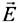, the magnetic field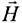, the electric flux density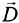and the magnetic induction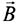. Only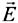and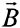vectors produce actions by which it is possible to measure the electromagnetic field. The vectors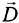and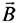are connected to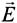and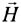vectors by the following linear relations: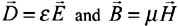The coefficients µ and ¼ are respectively the permittivity and the magnetic permeability of the medium.

In the absence of charged particles, they are linked by Maxwell's equations: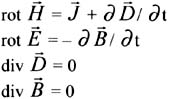Their resolution brings us to the Helmholz equation for each vector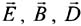and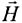. The vector(wherebelongs to the unitor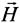) is then given by the following equation: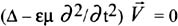where:

is the Laplacian operator: grad(div) - rot (rot)

In sinusoidal mode, the oscillations of the vectors E and H are propagated in space perpendicularly one to the other in the form of a wave (Figure 14.1) at velocity v = ( µ¼ ) ˆ’ 1/2 . The time interval between two equal successive elongations in direction and size is called the period . The space crossed by the wave during this time is the wavelength . It is given by the relation:where:

• f is the wave frequency,

• is the pulsation,

• T is the period.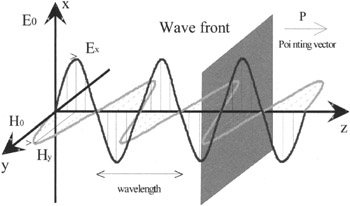Figure 14.1: Illustration of the propagation of an electromagnetic wave

The Pointing vector describes amplitude and direction of transported power flux.

Electromagnetic waves are generated by oscillating electric circuits, electric vibrations of dipoles or by electronic tubes. Their spectrum is very broad: we have ELF ( » >100 km), VLF (10< » <100 km), LF (1< » <10 km), MF (100< » <1000 m), HF (10< » <100 m), VHF (1< » <10 m), UHF (10< » <100 cm), SHF (1< » <10 cm), EHF (1< » <10 mm), sub-EHF (0.1< » <1 mm), infrared (0,8< » <5 ¼ m) or luminous waves (4000 …< » <8000 …).

The propagation conditions (atmospheric, terrestrial influence), the system considered (antenna, etc) as well as the associated services depend on the frequency band used. An antenna has high efficiency if its size is about or a half-length of the wavelength. This criterion can consequently determine the frequency band used of the designed system or communicating object.

For instance, the millimeter band (EHF band) is characterised by the following elements: at atmospheric level, propagation is sensitive to attenuation due to oxygen , water vapor, hydrometeors (rain, snow, hail etc), clouds, fog, dust or smoke, to hydrometeor scattering and scintillations arising from fluctuations of the refraction index. It is also dependent upon shadowing effects mainly due to buildings and trees. The antennas used will be in the form of small parabolas . The services will turn around with line of sight proximity communications (fixed or mobile), teledetection by satellite etc.

The use of Maxwell's equations is generally very difficult to analyse with problems arising and we often have recourse to approximation methods . The size of the irregularity d, met during propagation, compared to the wavelength (connected to the frequency) plays an important role. The three following cases are considered:

• d/ » < 1: irregularities are very small compared to the wavelength; statistical methods are then applied. The physical phenomena concerned will be primarily attenuation resulting from absorption and scattering.

• d/ » ‰ˆ 1: irregularities are of the same order as the wavelength; approximations are then not possible ( resonance zone).

• d/ » > 1: irregularities are very large compared to the wavelength: asymptotic methods based on optics and rays theory are applicable . The physical phenomena concerned will be primarily reflection, transmission, diffraction , etc.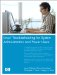Linux Troubleshooting for System Administrators and Power Users
ISBN: N/A
EAN: 2147483647
Year: 2005
Pages: 191

Similar book on Amazon